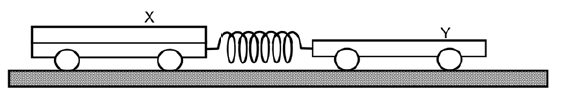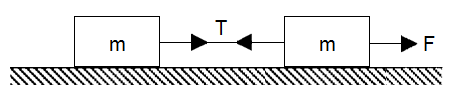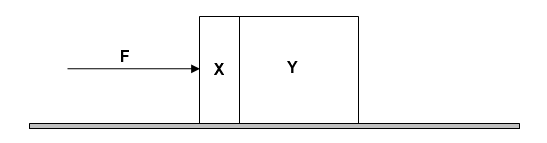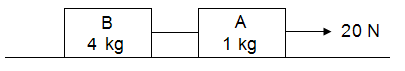Chapter 3 Forces Part 5 - Newton's Third Law
Newton's third law is: For every action, there is an equal and opposite reaction. The statement means that in every interaction, there is a pair of forces acting on the two interacting objects. The size of the forces on the first object equals the size of the force on the second object.
Name *
Class *
Index Number *
Trolley X and trolley Y are joined together by a compressed spring. The mass of trolley X is twice that of trolley Y. Both trolleys are initially at rest. When the trolleys are released, trolley Y accelerates to the right at 1.0 m/s². What is the acceleration of trolley X?
1 pointTwo equal masses m are connected by an inextensible string and move across a smooth frictionless horizontal surface with uniform acceleration, when pulled by a force F. The tension T in the connecting string is *
1 pointTwo blocks X and Y, of masses m and 4m respectively, are accelerated along a smooth horizontal surface by a force F applied to the block X as shown. What is the magnitude of the force exerted by block X on block Y during this acceleration? *
1 pointA force of 20 N acts on block A and block B of mass 1 kg and 4 kg respectively as shown. Given that the floor is smooth, what is acceleration of block A? *
1 pointA force of 20 N acts on block A and block B of mass 1 kg and 4 kg respectively as shown. 15. Given that the floor is smooth, what is the tension of the string which is between block A and block B? *
1 point